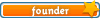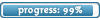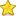Welcome

It is highly recommended that you have Javascript enabled; many features will not work unless you do. The recommended browsers are Firefox and Chrome; the board is also NOT mobile-friendly.

If you were referred by someone (ex. me, Jessica) please put their username in the referral box on the registration page. Ask them if you don't know their username.

If you are visiting for TESTING PURPOSES ONLY, this is the test account information:

Test

Select a forum to post in:

## The Theory of Signal Detection

Miscellaneous topics

Moderators: Jessica, Teachers

### The Theory of Signal Detection

The theory of signal detection was developed by mathematicians and engineers in the 1950's working in the fields of mathematical statistics and electronic communications. Signal detection deals with the detectability of signals and controlling the criterion that are used for the detection of signals. Early on, it became apparent that this theory has application to psychophysics because the observer's criterion affects the judgements they make. The theory of signal detection allows for the ability to separate the effects of the stimulus detectability from the observer's criterion in sensory experiments.

The following figure will be used to explain the key concepts we will need for signal detection theory:The subject's task is to detect a signal which is presented along some sensory continuum. For example, the sensory continuum in the case of the experiment of Hecht, Schlaer and Pirenne, is a visual continuum of flash intensity. Present in the observers nervous system is noise that may come from a variety of sources such as spontaneous isomerizations and spontaneous neural discharge. When a signal, a flash in this case, is presented to the subject, in order to detect the flash, the subject must discriminate the signal which is added to the inherant noise from the noise alone. We think of the noise as having a distribution; at any point in time the noise has a value that varies from a mean level. We will assume here that the noise distribution is normal. When a signal is added to the noise, the distribution is shifted to the right along the sensory continuum. Again we will assume that the signal + noise distribution is normally distributed and that it has the same standard deviation as the noise distribution alone. We can normalize these distributions (to simplify and standardize the math involved) so that the mean of the noise distribution is zero and the standard deviations of both distributions are 1.

When a signal+noise distribution (SN) is detectably different (let's assume we know the detectible difference, for now) from the noise distribution (N) the two distributions are separated by a distance called d' (d-prime). d' is a sensitivity index which is the distance of the mean of theSN distribution from the N distribution when the N distribution has a mean equal to zero and both distributions have a standard deviation of one.

When a subject is presented with the signal at any particular time, the signal will fall along the sensory continuum according to the SN distribution. The subject will base his judgement of detection of the signal according to some criterion along the sensory continuum. If no signal is presented during a trial the subject is still subject to an event at that time along the sensory continuum which has a probability associated with the N distribution. For any particular trial, the sensory event (which may be the result of a signal presentation or no signal presentation) is above the criterion level the subject will report seeing the flash. If the sensory event is below the criterion, he will report not seeing the flash.

Let's assume the subjects criterion is located at the point shown in the figure above. If you present the subject with multiple trials in which the signal is presented or not presented there will be a probability associated with the subjects response due to the distributions of the N and SN. These probabilities can be summarized in a conditional probability matrix. The rows of the matrix represent the presence or absence of a signal and the columns represent the subjects response.

If the subject says he saw the signal ("yes") when it was present, this is called a hit.
If the subject says he didn't see the signal ("no") when it was present, this is called a miss.
If the subject says he saw the signal ("yes") when it was absent, this is called a false alarm.
If the subject says he didn't see the signal ("no") when it was absent, this is called a correct rejection.The notation P(Y|SN) means the probability of a yes response given the presentation of the signal and P(N|N) means the probability of a no response given that the signal was absent.

So in the example presented here the table would look like this:So for example, when the signal is not present, there will be a false alarm rate of 8%. Notice that the probability sums to 1.0 reading across the table.
• 0Jessica
Board Owner
Topic AuthorSapphirePosts: 3,474
Topics: 1,244
Articles: 30
Joined: December 22nd, 2010, 8:04 pm